# The COUNTREG Procedure

### DISPMODEL Statement

• DISPMODEL dependent-variable<dispersion-related regressors>;

The DISPMODEL statement names the variables that are used to model dispersion. This statement is ignored unless you specify DIST=CMPOISSON in the MODEL statement. The dependent-variable in the DISPMODEL statement must be the same as the dependent-variable in the MODEL statement.

The dependent-variables that appear in the DISPMODEL statement are directly used to model dispersion. Each of these q variables has a parameter to be estimated in the regression. For example, let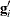be the ith observation’s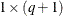vector of values of the q dispersion explanatory variables (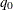is set to 1 for the intercept term). Then the dispersion is a function of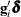, where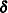is the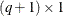vector of parameters to be estimated, the dispersion model intercept is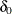, and the coefficients for the q dispersion covariates are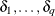. If you specify DISP=CMPOISSON in the MODEL statement but do not include a DISPMODEL statement, then only the intercept termis estimated. The “Parameter Estimates” table in the displayed output shows the estimates for the dispersion intercept and dispersion explanatory variables; they are labeled with the prefix "Disp_". For example, the dispersion intercept is labeled "Disp_Intercept". If you specify Age (a variable in your data set) as a dispersion explanatory variable, then the “Parameter Estimates” table labels the corresponding parameter estimate "Disp_Age". The following statements fit a Conway-Maxwell-Poisson model by using the covariates SEX, ILLNESS, and INCOME and by using AGE as a dispersion covariate:

   proc countreg data=docvisit;
model doctorvisits=sex illness income / dist=cmpoisson;
dispmodel doctorvisits ~ age;
run;